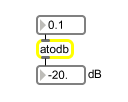# atodb

Convert linear amplitude to a deciBel value

## Description

atodb converts any given linear amplitude value to its corresponding deciBel value.

None.

## Messages

 bang The most recently calculated deciBel value is sent out the outlet. int linear-amplitude [int] A linear amplitude value. The corresponding gain/attenuation in deciBels is sent out the outlet. float linear-amplitude [float] A linear amplitude value. The corresponding gain/attenuation in deciBels is sent out the outlet. list list of gain/attention values [list] A list of linear amplitude values. Corresponding gain/attenuation values in deciBels for each list item are sent out the outlet. set linear-amplitude [float] The message set followed by a linear amplitude value will set the next value to be calculated into deciBels without sending anything out the outlet.

## Output

float: The gain or attenuation from unity gain, expressed in deciBels.
int: The gain or attenuation from unity gain, expressed in deciBels.

## Examples